# Identify the Odd One Out using Direction, Position and Number

In this worksheet, students will identify an image that does not fit in with the rest of the group. The focus will be on direction, position and number, so students will need to look at where shapes are, which direction they are facing, and how many there are.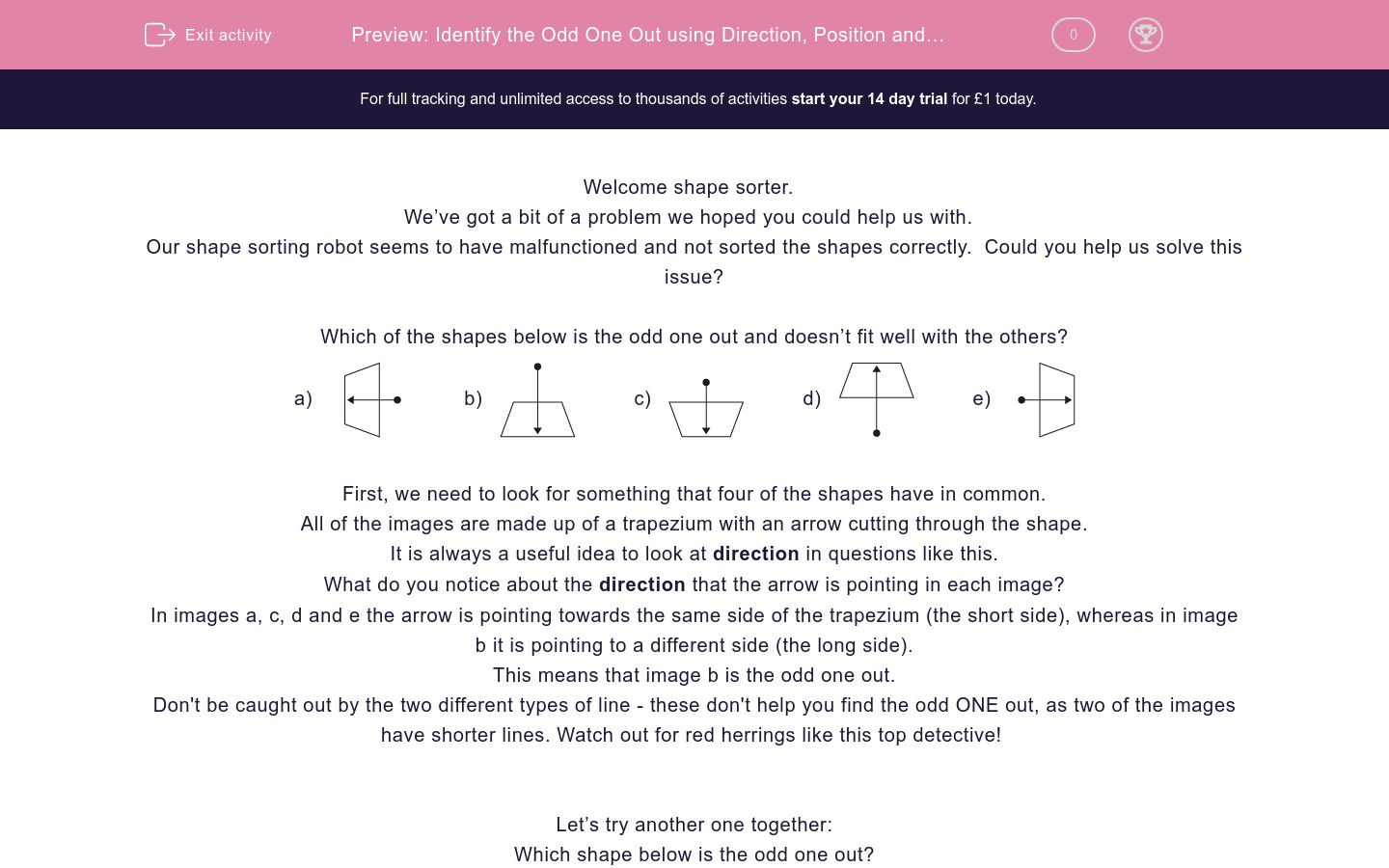Key stage:  KS 2

Curriculum topic:   Spatial and Non-Verbal Reasoning

Curriculum subtopic:   Odd One Out

Difficulty level:### QUESTION 1 of 10

Welcome shape sorter.

We’ve got a bit of a problem we hoped you could help us with.

Our shape sorting robot seems to have malfunctioned and not sorted the shapes correctly.  Could you help us solve this issue?

Which of the shapes below is the odd one out and doesn’t fit well with the others?

a)b)c)d)e)First, we need to look for something that four of the shapes have in common.

All of the images are made up of a trapezium with an arrow cutting through the shape.

It is always a useful idea to look at direction in questions like this.

What do you notice about the direction that the arrow is pointing in each image?

In images a, c, d and e the arrow is pointing towards the same side of the trapezium (the short side), whereas in image b it is pointing to a different side (the long side).

This means that image b is the odd one out.

Don't be caught out by the two different types of line - these don't help you find the odd ONE out, as two of the images have shorter lines. Watch out for red herrings like this top detective!

Let’s try another one together:

Which shape below is the odd one out?

a)b)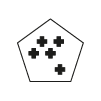c)d)e)First, we need to look for something that four of the shapes have in common.

In questions like this, counting is really important. What could we count?

We have two things to count here: the number of mini shapes and the number of sides of the main shape.

Image a is made up of a 3-sided shape with 3 crosses inside.

Image b is a 5-sided shape with 5 crosses inside.

Image c is a 4-sided shape with 4 crosses inside.

Image d is a 6-sided shape with 5 crosses inside.

Image e is a 4-sided shape with 4 crosses inside.

This means that image d is the odd one out as the number of sides of the main shape does not match the number of mini shapes inside, unlike the other options.

It’s now your turn to identify the odd image.

Remember to look at the direction and position of shapes within the image and always see if there is something you can count.

Good luck shape sorter!

Which of the images below is the odd one out?

a)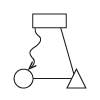b)c)d)e)Which of the images below is the odd one out?

a)b)c)d)e)Which of the images below is the odd one out?

a)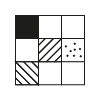b)c)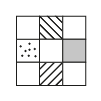d)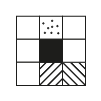e)Which of the images below is the odd one out?

a)b)c)d)e)Which of the images below is the odd one out?

a)b)c)d)e)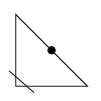Which of the images below is the odd one out?

a)b)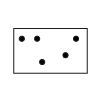c)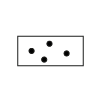d)e)Which of the images below is the odd one out?

a)b)c)d)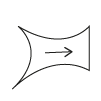e)Which of the images below is the odd one out?

a)b)c)d)e)Which of the images below is the odd one out?

a)b)c)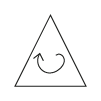d)e)Which of the images below is the odd one out?

a)b)c)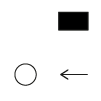d)e)• Question 1

Which of the images below is the odd one out?

c)EDDIE SAYS
This is a direction-focused question. Where is the squiggly line with an arrow pointing to in each image? Remember that arrows often POINT towards clues. In options a, d, b and e the squiggly line is pointing to the circle. In option c the squiggly lines is pointing to the rectangle. This is pointing in the wrong direction, so makes option c the odd one out. Always look at where the arrows are pointing to help you... it's an important POINT to remember.
• Question 2

Which of the images below is the odd one out?

d)EDDIE SAYS
This is a number-themed question. It's always important to see what we can COUNT in these questions. Here we can choose between triangles and lines. In options a, b, c and e there are a matching number of triangles and lines. For example, in option a there are 3 triangles and 3 lines. Option d has 3 triangles and 2 lines. The number of triangles and lines do not match in option d like they do in the others, which makes it the odd one out.
• Question 3

Which of the images below is the odd one out?

e)EDDIE SAYS
It's another counting question. All of the squares have the same number of inner squares, but we still have a few options on what to count. Each square has a combination of shaded, blank, dotty and lined squares. To answer this question, we needed to count the total number of decorated squares in each image. In options a, b, c and d there are 4 decorated squares in total, whereas in option e there are 5. This makes option e the odd one out for being too greedy with its decoration!
• Question 4

Which of the images below is the odd one out?

c)EDDIE SAYS
This is another number question. There is a relationship between the number of sides of each shape and the number of lines running through it. Can you spot what it is? Option a has 4 sides and 4 lines running through it. Option b has 3 sides and 3 lines. This pattern of the number of sides and lines matching works for all the options except for option c, which makes it the odd one out.
• Question 5

Which of the images below is the odd one out?

e)EDDIE SAYS
This was a tricky one! We have to consider position here. The black circles are all on the edges of the triangles so that doesn't help. Let's look at the position of the lines. The small lines in options a, b, c and d all cross over the acute angle of the triangle, whereas in option e the line crosses over the right angle. This makes option e the odd one out. Did you know that an acute angle is an angle which is less than 90 degrees?
• Question 6

Which of the images below is the odd one out?

b)EDDIE SAYS
This is a number relationship question. There is a connection between the numbers of sides of the main shape and the number of black balls. Option a has 3 sides and 3 black balls. Option c has 4 sides and 4 black balls. Can you spot the unbalanced image? Unfortunately option b has 4 sides but 5 black balls so it does not follow the same rule. This makes option b the odd one out.
• Question 7

Which of the images below is the odd one out?

e)EDDIE SAYS
It's time to think directionally now detective! All of these shapes are quadrilaterals made up of three curved sides and one straight side. In options a, b, c and d the arrow is pointing to the straight side of the shape. In option e the arrow is pointing to a curved side, making it the odd one out.
• Question 8

Which of the images below is the odd one out?

c)EDDIE SAYS
Back to a numbers question now. There is a relationship between the number of dashes and the number of circles on each main line. In options a, b, d and e there are a matching number of circles and dashes. For example, option b has 3 lines and 3 circles. This rule doesn't apply to image c, as it has 3 circles and only 2 dashes. This makes option c the odd one out.
• Question 9

Which of the images below is the odd one out?

d)EDDIE SAYS
Did you let the arrow POINT you to the answer top detective? This question focuses on direction. In options a, b, c and e the arrow is pointing in a clockwise direction, but in option d it is pointing anti-clockwise. This makes option d the odd one out. Remember clockwise means moving in the same direction as the hands on the face of a clock.
• Question 10

Which of the images below is the odd one out?

c)EDDIE SAYS
Where is the arrow pointing here? In four of the five options, the arrow is pointing to a black shape, but in option c it is pointing to a white shape. This makes option c the odd one out. Great work picking up on all the clues to solve these questions detective, you can't let anything slip past you!
---- OR ----

Sign up for a £1 trial so you can track and measure your child's progress on this activity.

### What is EdPlace?

We're your National Curriculum aligned online education content provider helping each child succeed in English, maths and science from year 1 to GCSE. With an EdPlace account you’ll be able to track and measure progress, helping each child achieve their best. We build confidence and attainment by personalising each child’s learning at a level that suits them.

Get started